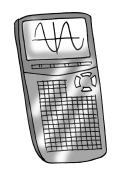### Home > PC3 > Chapter 9 > Lesson 9.1.3 > Problem9-55

9-55.

Now practice using a calculator to compute matrix products. Use matrices $B$ and $E$ from problems 9-27 and 9-28, shown at right.1. Enter $E$ and $B$ into a graphing calculator.

2. Which product, $EB$ or $BE$, should be computed to make sense in this situation. Use a calculator to compute this product. What does this product represent?

Multiplying a $3 \times 2$ matrix by a $2 \times 4$ matrix results in a $3 \times 4$ matrix.
Therefore, which of the following products makes sense and what is the result?

employees $\times$ bouquet styles by bouquet styles $\times$ flowers

bouquet styles $\times$ flowers by employees $\times$ bouquet styles

 $\boldsymbol E$ Bouquet Styles Employees ABC #1   #2  #3$\begin{bmatrix} {6} & { 4 } & { 7} \\ { 4 } & { 8 } & { 5 }\\ { 5 } & { 6 } & { 6 } \end{bmatrix}$
 $\boldsymbol B$ Flowers Bouquet Styles ABC L    R   D$\begin{bmatrix} {5} & { 4 } & { 3} \\ { 4 } & { 3 } & { 3 }\\ { 4 } & { 6 } & { 6 } \end{bmatrix}$